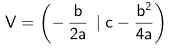Quadratic function  »If you want to determine the
vertex of a parabola using the vertex formula,
you can do this as follows:
 1. determine p and q from the standard form of the parabola equation y = ax2 + bx + c = 0 2. calculate the x-coordinate and then the y-coordinate. Use the vertex formula for this:Click on  new  to create a new problem.
Can you top 295 points?

Determine a, b, c and the vertex V!
 V = (, )

realmath.de

... more than just practicing